Getting StartedMesh and Surface Plots

MATLAB defines a surface by the z-coordinates of points above a grid in the x-y plane, using straight lines to connect adjacent points. The `mesh` and `surf` plotting functions display surfaces in three dimensions. `mesh` produces wireframe surfaces that color only the lines connecting the defining points. `surf` displays both the connecting lines and the faces of the surface in color.

The figure colormap and figure properties determine how MATLAB colors the surface.

Visualizing Functions of Two Variables

To display a function of two variables, z = f (x,y),

• Generate `X` and `Y` matrices consisting of repeated rows and columns, respectively, over the domain of the function.
• Use `X` and `Y` to evaluate and graph the function.

The `meshgrid` function transforms the domain specified by a single vector or two vectors `x` and `y` into matrices `X` and `Y` for use in evaluating functions of two variables. The rows of `X` are copies of the vector `x` and the columns of `Y` are copies of the vector `y`.

Example -- Graphing the sinc Function

This example evaluates and graphs the two-dimensional sinc function, sin(r)/r, between the x and y directions. `R` is the distance from the origin, which is at the center of the matrix. Adding `eps` (a MATLAB command that returns a small floating-point number) avoids the indeterminate 0/0 at the origin:

• ```[X,Y] = meshgrid(-8:.5:8);
R = sqrt(X.^2 + Y.^2) + eps;
Z = sin(R)./R;
mesh(X,Y,Z,'EdgeColor','black')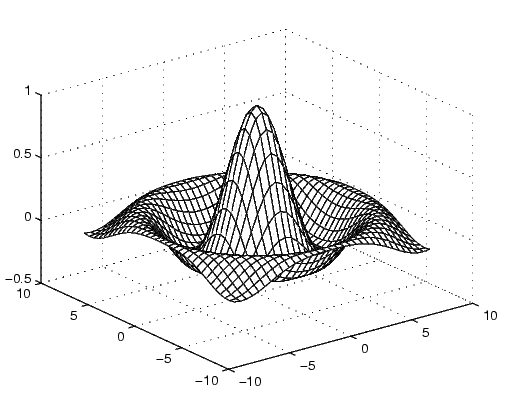```

By default, MATLAB colors the mesh using the current colormap. However, this example uses a single-colored mesh by specifying the `EdgeColor` surface property. See the `surface` reference page for a list of all surface properties.

You can create a mesh with see-through faces by disabling hidden line removal:

• ```hidden off
```

See the `hidden` reference page for more information on this option.

Example -- Colored Surface Plots

A surface plot is similar to a mesh plot except that MATLAB colors the rectangular faces of the surface. The color of each faces is determined by the values of `Z` and the colormap (a colormap is an ordered list of colors). These statements graph the sinc function as a surface plot, specify a colormap, and add a color bar to show the mapping of data to color:

• ```surf(X,Y,Z)
colormap hsv
colorbar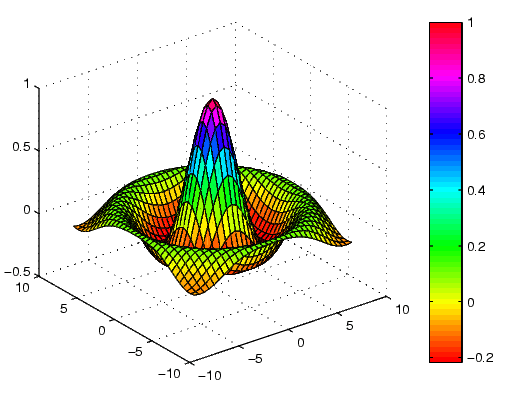```

See the `colormap` reference page for information on colormaps.

Transparent Surfaces

You can make the faces of a surface transparent to a varying degree. Transparency (referred to as the alpha value) can be specified for the whole object or can be based on an alphamap, which behaves in a way analogous to colormaps. For example,

• ```surf(X,Y,Z)
colormap hsv
alpha(.4)
```

produces a surface with a face alpha value of 0.4. Alpha values range from 0 (completely transparent) to 1 (not transparent).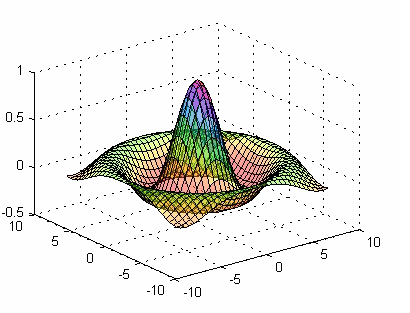Surface Plots with Lighting

Lighting is the technique of illuminating an object with a directional light source. In certain cases, this technique can make subtle differences in surface shape easier to see. Lighting can also be used to add realism to three-dimensional graphs.

This example uses the same surface as the previous examples, but colors it red and removes the mesh lines. A light object is then added to the left of the "camera" (the camera is the location in space from where you are viewing the surface):

• ```surf(X,Y,Z,'FaceColor','red','EdgeColor','none')
camlight left; lighting phong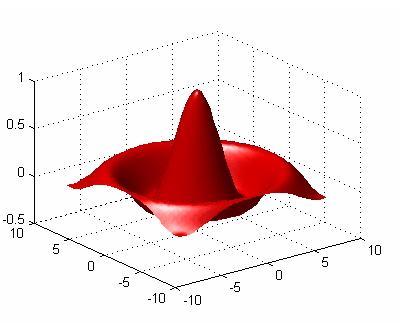```

Manipulating the Surface

The figure toolbar and the camera toolbar provide ways to explore 3-D graphics interactively. Display the camera toolbar by selecting Camera Toolbar from the figure View menu.

The following picture shows both toolbars with the Rotate 3D tool selected.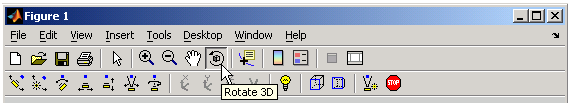These tools enables you to move the camera around the surface object, zoom, add lighting, and perform other viewing operations without issuing commands.

The following picture shows the surface viewed by orbiting the camera toward the bottom using Rotate 3D. A scene light has been added to illuminate the underside of the surface, which is not lit by the light added in the previous section.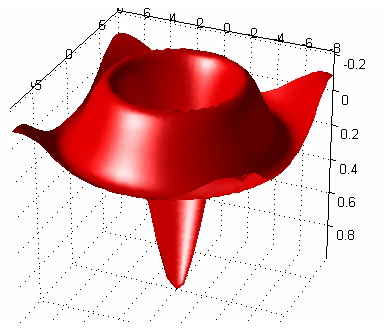For More Information    See Lighting as a Visualization Tool and View Control with the Camera Toolbar in the MATLAB documentation for information on these techniques.Saving Figures Images